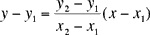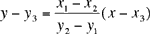Quandaries and Queries Hi, Given a line segment L(x1,y1) to (x2,y2) and a Point P(x3,y3). I need the formula to find a point on the line L that is closest to the point (x3,y3). Please help! Meenakshi. Hi Meenakshi, First write the equation of the line through (x1,y1) and (x2,y2).The line through (x3,y3) and perpenducular to the above line isThe point you want is the point of intersection of these two lines. Penny Go to Math Central